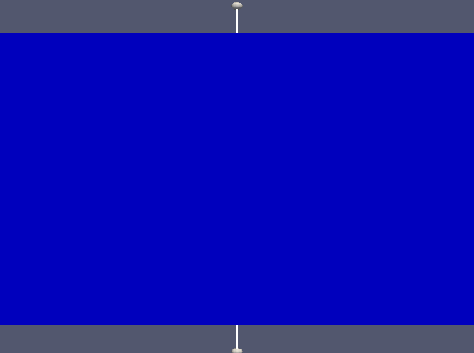# Capillary pressure influence

As it is known, in multiphase flow it could exist a  difference of pressure between phases present in the porous media. The difference of pressure is called the capillary pressure and is due to the existence of surface tension between the phases involved.

When we take into account the capillary pressure, we have a gradient of this pressure added to the conservation equations.

# Capillary pressure modeling

In the literature  exists several empirical formula of capillary pressure present in a porous media.

To understand the effect of the capillary pressure on the saturation, we did our simulation with the following simple formula as a first approach :

$P_c=C(S_e)^{-\alpha}$

where :

•  $P_c$ is the capillary pressure
•  $C$ and $\alpha$ are constant
•  $S_e$ is the effective saturation in liquid.

Se is defined by :• where $S_{min}$ and $S_{max}$ are the minimal and the maximal saturation of the porous media.

# Simulations

We did all the  simulations in this part with the same parameters as in 3.3.1 and by taking into accounting the presence of a capillary pressure with $\alpha=0.2$ and $C=10.10^3 Pa$.

We are going to compare the oil saturation over a vertical line from the domain with capillary pressure and without capillary pressure.

Plot line :# Simulations with capillary pressure and qwell=0

In order to observe the effect of the capillary pressure on the saturation we set the source term equal to 0.

Oil saturation t=0 sOil saturation t=130000 sWe observe that saturation is increased in the boundary region between oil and air. Indeed the water goes up due to the capillary pressure, after certain time the water stop going up due the balance by gravity

# Simulations with capillary pressure with pumping

We compare the influence the case with capillary and the case without capillary pressure.

Saturation without $P_c$, time=100000 sSaturation with $P_c$,  time=100000 sWe observe that the capillary pressure allows the oil to go up and help to the pumping .
Moreover we know that pumping create a lack of oil which increases the influence of the capillary pressure.